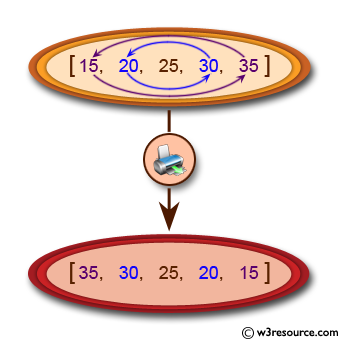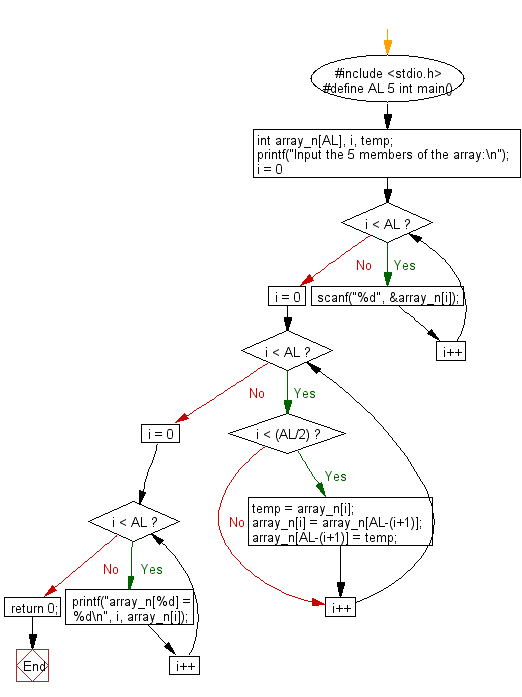﻿ C Program: Print the elements of an modified array - w3resource

# C Exercises: Print the elements of an modified array

## C Basic Declarations and Expressions: Exercise-51 with Solution

Write a C program to read an array of length 6, change the first element by the last, the second element by the fifth and the third element by the fourth. Print the elements of the modified array.

Pictorial Presentation:Sample Solution:

C Code:

``````#include <stdio.h>
#define AL 5
int main() {
// Define the size of the array
int array_n[AL], i, temp;

// Prompt user to input 5 elements of the array
printf("Input the 5 members of the array:\n");
for(i = 0; i <  AL; i++) {
scanf("%d", &array_n[i]);
}

// Swap the first half of the array with the second half
for(i = 0; i < AL; i++) {
if(i < (AL/2)) {
temp = array_n[i];
array_n[i] = array_n[AL-(i+1)];
array_n[AL-(i+1)] = temp;
}
}

// Print the modified array
for(i = 0; i < AL; i++) {
printf("array_n[%d] = %d\n", i, array_n[i]);
}

return 0;
}
``````

Sample Output:

```Input the 5 members of the array:
15
20
25
30
35
array_n = 35
array_n = 30
array_n = 25
array_n = 20
array_n = 15
```

Flowchart:C programming Code Editor:

What is the difficulty level of this exercise?

Test your Programming skills with w3resource's quiz.

﻿